# PHP – MYSQL : sum() operation

• Last Updated : 01 Oct, 2021

Problem Statement:

In this article, we are going to perform sum() aggregate operation on our database using PHP with xampp server.

So we are considering the food_order database and perform database sum() operation.

Requirements:

xampp

Introduction:

PHP stands for hypertext preprocessor which is a server-side scripting language connect with databases.

it is connected with MySQL to perform manipulations in a database.

MySQL is a database query language for managing databases.

• sum()

sum() function is an aggregation operation used to add the particular column based on the given condition.

Syntax:

```SELECT SUM(column1),column2,...column1 from
table_name;```

It can be also used with GROUP BY & HAVING clause.

Example query:

Consider the table: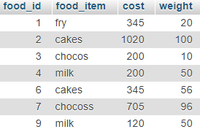Find the total cost of food items

`SELECT  SUM(cost) from food;`

Output:

`Total cost: 2935`

Find the total weight of food items

`SELECT  SUM(weight) from food;`
`Total weight: 382`

Find the total cost of food items with respect to items

```SELECT food_item, SUM(cost) FROM food GROUP BY food_item;
Total cakes = 1365
Total chocos = 200
Total chocoss = 705
Total fry = 345
Total milk = 320```

Approach

• Create  a database
• Create a table
• PHP code to perform sum() operation

Steps

• Start xampp server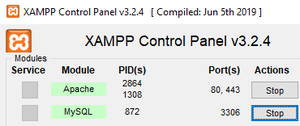• Create a database named geek and create a table named food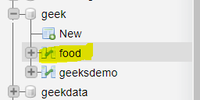Table structure: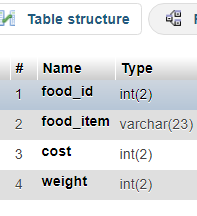Refer this for how to insert records in xampp

https://www.geeksforgeeks.org/performing-database-operations-in-xampp/

Finally, the food table contains• PHP code to perform sum function

form.php

After writing code type “localhost/form.php” to view the output in the browser.

## PHP

 `````"``; ``echo` `"sum() aggregate  Demo "``; ``echo``""``;``echo` `"

"``;``echo` `" Find the total cost of food items "``;``echo` `"

"``;``//sql query``\$sql` `= ``"SELECT  SUM(cost) from food"``;``\$result` `= ``\$conn``->query(``\$sql``);``//display data on web page``while``(``\$row` `= mysqli_fetch_array(``\$result``)){``    ``echo` `" Total cost: "``. ``\$row``[``'SUM(cost)'``];``    ``echo` `"
"``;``}` `echo` `"

"``;``echo` `" Find the total weight of food items "``;``echo` `"

"``;``//sql query``\$sql` `= ``"SELECT  SUM(weight) from food"``;``\$result` `= ``\$conn``->query(``\$sql``);``//display data on web page``while``(``\$row` `= mysqli_fetch_array(``\$result``)){``    ``echo` `" Total weight: "``. ``\$row``[``'SUM(weight)'``];``    ``echo` `"
"``;``}`  `//close the connection` `\$conn``->close();``?>`````

Output: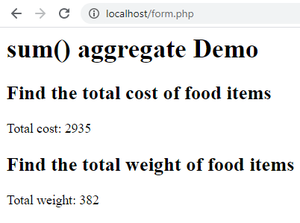Example: sum() with group by clause

form1.php

## PHP

 `````"``; ``echo` `"sum() aggregate with group by Demo "``; ``echo``""``;``echo` `"

"``;``echo` `"`` ``";echo "``

";``//sql query``\$sql` `= ``"SELECT food_item, SUM(cost) FROM food GROUP BY food_item"``;``\$result` `= ``\$conn``->query(``\$sql``);``//display data on web page``while``(``\$row` `= mysqli_fetch_array(``\$result``)){``    ``echo` `"Total "``. ``\$row``[``'food_item'``]. ``" = "``. ``\$row``[``'SUM(cost)'``];``      ``echo` `"
"``;``}``//close the connection` `\$conn``->close();``?>`````

Output: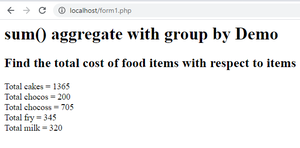My Personal Notes arrow_drop_up